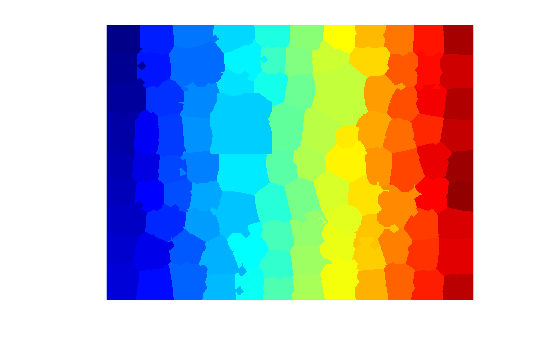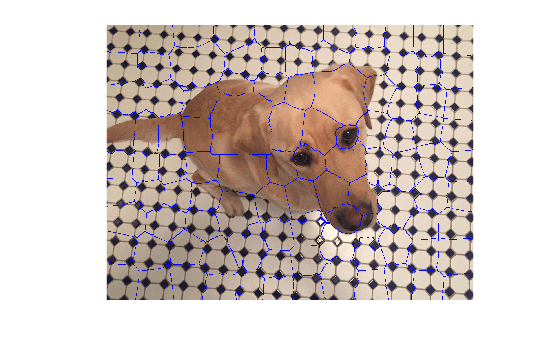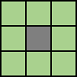Find region boundaries of segmentation

## Syntax

``mask = boundarymask(L)``
``mask = boundarymask(BW)``
``mask = boundarymask(___,conn)``

## Description

example

````mask = boundarymask(L)` computes a mask that represents the region boundaries for the input label matrix `L`. The output, `mask`, is a logical image that is `true` at boundary locations and `false` at non-boundary locations.```
````mask = boundarymask(BW)` computes the region boundaries for the input binary image `BW`.```
````mask = boundarymask(___,conn)` computes the region boundaries using a connectivity specified by `conn`. ```

## Examples

collapse all

Read an image into the workspace.

`I = imread('kobi.png');`

Create a superpixel representation of the image, returned as a label matrix.

`L = superpixels(I,100);`

Display the label matrix.

`imshow(label2rgb(L))`Find the region boundaries of the label matrix.

`mask = boundarymask(L);`

Display the boundary mask over the original image by using the `labeloverlay` function. The region boundaries of the label matrix appear as 1-pixel wide cyan lines.

`imshow(labeloverlay(I,mask,'Transparency',0))`## Input Arguments

collapse all

Label matrix, specified as a 2-D numeric matrix of nonnegative numbers or a 2-D logical matrix.

Data Types: `single` | `double` | `int8` | `int16` | `int32` | `uint8` | `uint16` | `uint32` | `logical`

Binary image, specified as a numeric or logical matrix of the same size as `L`.

Data Types: `single` | `double` | `int8` | `int16` | `int32` | `int64` | `uint8` | `uint16` | `uint32` | `logical`

Pixel connectivity, specified as `4` or `8`.

Value

Meaning

Two-Dimensional Connectivities

`4`

Pixels are connected if their edges touch. The neighborhood of a pixel are the adjacent pixels in the horizontal or vertical direction.Current pixel is shown in gray.

`8`

Pixels are connected if their edges or corners touch. The neighborhood of a pixel are the adjacent pixels in the horizontal, vertical, or diagonal direction.Current pixel is shown in gray.

Data Types: `single` | `double` | `int8` | `int16` | `int32` | `uint8` | `uint16` | `uint32`

## Output Arguments

collapse all

Rasterized grid of region boundaries, specified as a 2-D logical matrix of the same size as the input image. A pixel in `mask` is `true` when the corresponding pixel in the input image with value P has a neighboring pixel with a different value than P.

Data Types: `logical`

## Version History

Introduced in R2016a